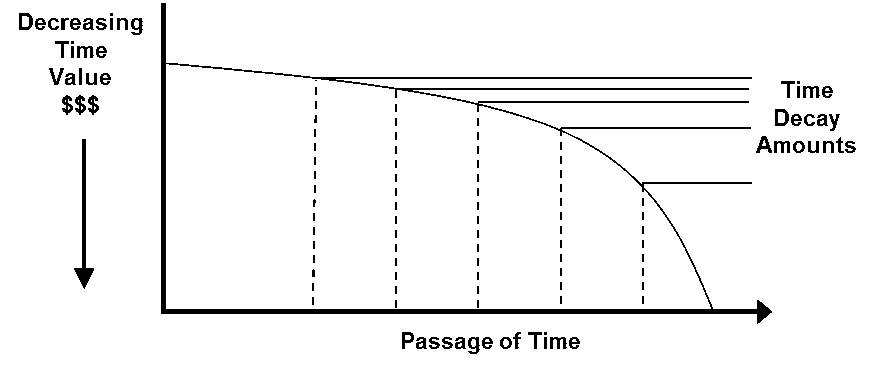## Put Option Profit Formula

A put option is a contract that gives the owner the right, but not the obligation, to sell an agreed-upon number of an underlying security (e.g. shares) by a specific date and to sell the underlying security at a pre-determined price, called the strike price.

As the price of the underlying security changes, it will affect the price of the put option and thus the potential profit that the put holder can enjoy. So how is a put option profit calculated? In order to understand how profitable a put option is, you must first understand the concept of intrinsic value.

Intrinsic value is the difference between the current market price of the underlying asset and the strike price of the option. Whenever the strike price of a put is greater than the market price of the underlying asset, it is considered to be in-the-money. This is important to remember, as only in-the-money options have an intrinsic value.

The second concept to understand is the time value of an option. The time value of an option decays as the option approaches the expiration date.Image source: CME Group

What this means is that as the expiration date gets closer, the more the value of the option is attributed to the intrinsic value. Finally, when the option expires, it has no time value component, so its value is completely determined by the intrinsic value.

So how do you calculate the intrinsic value of an option? We’ll demonstrate how, using an example. Suppose you purchased a \$20 put option for company ABC at a strike price of \$75. If the stock of ABC is currently trading at \$70, you would enjoy an intrinsic value of \$15.

This is calculated by taking the price of the put option (\$20) and subtracting the difference between the strike price and the current underlying price (\$75 – \$70 = \$5). The remaining 5 points are simply the premium that is attributable to time value (which will decline as the option approaches expiration). Since each option contract is for 100 shares, this means that the total cost of the put option would be \$2,000 (which is 100 shares x the \$20 purchase price).

Disclaimer: The information above is for educational purposes only and should not be treated as investment advice. The strategy presented would not be suitable for investors who are ...

more
How did you like this article? Let us know so we can better customize your reading experience. Users' ratings are only visible to themselves.